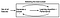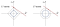# Comparing Linear Regression Models: Lasso vs Ridge

Recently, I learned about making linear regression models and there were a large variety of models that one could use. When looking at a subset of these, regularization embedded methods, we had the LASSO, Elastic Net and Ridge Regression. For right now I’m going to give a basic comparison of the LASSO and Ridge Regression models.

## Regularization Embedded Models

So Embedded methods are models that learn which features best contribute to the accuracy of the model while the model is running. The methods we are talking about today regularize the model by adding additional constraints on the model to aim toward lowering the size of the coefficients and in turn making a less complex model. This would help against over-fitting your model, where it would perform much better on the training set than it would on the testing set. We will see that while both the LASSO and Ridge Regression models add constraints, the resulting coefficients and their sizes differ and the approach is a bit different.Embedded Method Process

## LASSO Model

The LASSO method aims to produce a model that has high accuracy and only uses a subset of the original features. The way it does this is by putting in a constraint where the sum of the absolute values of the coefficients is less than a fixed value. To that end it lowers the size of the coefficients and leads to some features having a coefficient of 0, essentially dropping it from the model. In this way, it is also a form of filtering your features and you end up with a model that is simpler and more interpretable.

The LASSO, however, does not do well when you have a low number of features because it may drop some of them to keep to its constraint, but that feature may have a decent effect on the prediction. It also does not do well with features that are highly correlated and one(or all) of them may be dropped when they do have an effect on the model when looked at together.Constraint Regions for LASSO(left) and Ridge Regression(right) -Wikipedia

## Ridge Regression

The Ridge Regression method was one of the most popular methods before the LASSO method came about. The idea is similar, but the process is a little different. The Ridge Regression also aims to lower the sizes of the coefficients to avoid over-fitting, but it does not drop any of the coefficients to zero. The constraint it uses is to have the sum of the squares of the coefficients below a fixed value. The Ridge Regression improves the efficiency, but the model is less interpretable due to the potentially high number of features.

It performs better in cases where there may be high multi-colinearity, or high correlation between certain features. This is because it reduces variance in exchange for bias. You also need to make sure that the number of features is less than the number of observations before using Ridge Regression because it does not drop features and in that case may lead to bad predictions.

## Concluding Thoughts

To summarize, LASSO works better when you have more features and you need to make a simpler and more interpretable model, but is not best if your features have high correlation. Ridge Regression works better when you have less features or when you have features with high correlation, but otherwise, in most cases, should be avoided due to higher complexity and lower interpretability(which is really important for practical data evaluation).

The point of this post is not to say one is better than the other, but to try to clear up and explain the differences and similarities between LASSO and Ridge Regression methods. As seen above, they both have cases where they perform better. There is also the Elastic Net method which is basically a modified version of the LASSO that adds in a Ridge Regression-like penalty and better accounts for cases with high correlated features.

Data Science student at Flatiron School

## More from Zawar Ahmed

Data Science student at Flatiron School